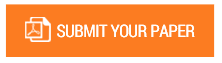Bayesian Analysis of Gross Domestic Product in Nigeria

Authors : M.A ADIO; O.U. ODUKOYA

Volume/Issue : Volume 7 - 2022, Issue 3 - March

Scribd : https://bit.ly/3LbbGeV

DOI : https://doi.org/10.5281/zenodo.6471750

The Gross Domestic Product (GDP) measures all commodities and services generated in the country, regardless of whether they are produced by domestic or foreign firms. It examines the country's economic growth, pattern, and rate. As a result, the focus of this research is on Nigeria's GDP rate. The data was subjected to exploratory data analysis (GDP rate).The data's minimum and maximum values were established. Easy fit was used to match the optimal distribution for the data set after the histogram and box plot were shown. In order to determine their respective posterior distributions, the Bayes theorem was also applied to both the conjugate and non-informative priors. The two priors' posterior means and standard deviations, as well as their credible intervals, were calculated.The results showed that the mean and standard deviation for the data were 1411.6 and 928.8775, with the minimum and maximum values to be 383 and 3567 respectively. The histogram showed that the data is positively skewed to the right, the box plot indicated the lower and the upper quartiles were 620 and 2187. The application of Bayes theorem to the data set, assumed a beta distribution for conjugate prior, while a uniform distribution was assumed for the Noninformative prior. The parameter values for the conjugate prior, the likelihood and the posterior distributions were Beta(4.21,7.39), Beta(5.07,10.83) and Beta(8.28 and 17.01) respectively. The posterior Mean (Bayes estimate) and Standard deviation as well as 95% credible interval for Beta prior were 0.32373, 0.0917 and [0.31, 0.34]. Also the Posterior mean, standard deviation as well as the 95% credible interval for the uniform prior were 0.3229, 0.114543 and [0.20836, 0.4374]. The posterior mean for the uniform prior was higher than the posterior mean for the conjugate prior, the credible interval for the conjugate prior was closer than the credible interval for the uniform prior, and the posterior standard deviation for the conjugate prior was higher than the posterior standard deviation for the uniform prior. As a result, the conjugate prior outperforms the uniform prior. Beta likelihood is recommended for fitting the rate of GDP in Nigeria.

Keywords : Beta distribution, Uniform Prior, Credible Interval, Bayes estimates, Posterior distribution.

#### CALL FOR PAPERS

Paper Submission Last Date
31 - December - 2023